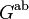# Group with unique Schur covering group

This article defines a group property: a property that can be evaluated to true/false for any given group, invariant under isomorphism
View a complete list of group properties
VIEW RELATED: Group property implications | Group property non-implications |Group metaproperty satisfactions | Group metaproperty dissatisfactions | Group property satisfactions | Group property dissatisfactions

## Statement

A group with unique Schur covering group is a group$G$ satisfying the following equivalent conditions:

1. It has a unique Schur covering group, i.e., given Schur covering groups$K_1,K_2$ of$G$ with covering maps$\pi_1: K_1 \to G$ and$\pi_2: K_2 \to G$, there is an isomorphism$\theta:K_1 \to K_2$ such that$\pi_1 = \pi_2 \circ \theta$.
2.$\operatorname{Ext}^1(G^{\operatorname{ab}},M(G)) = 0$ where$G^{\operatorname{ab}}$ is the abelianization of$G$ (and also identified with$H_1(G;\mathbb{Z})$) while$M(G)$ is the Schur multiplier of$G$ (and also identified with$H_2(G;\mathbb{Z})$). Note that for$G$ a finite group, this is equivalent to verifying that$G^{\operatorname{ab}}$ and$M(G)$ have relatively prime orders.

## Examples

The smallest example of a group$G$ where neither the abelianization nor the Schur multiplier is trivial, but the Schur covering group is still unique, is alternating group:A4, whose unique Schur multiplier is special linear group:SL(2,3).

In addition, we can take perfect groups and Schur-trivial groups as examples.

### Non-examples

The smallest non-example is the Klein four-group, which has two Schur covering groups (dihedral group:D8 and quaternion group). Note that in general the number of Schur covering groups is$|\operatorname{Ext}^1(G^{\operatorname{ab}},M(G))|$.

## Relation with other properties

### Stronger properties

Property Meaning Proof of implication Proof of strictness (reverse implication failure) Intermediate notions
perfect group the abelianization is trivial. (any Schur-trivial group that is not perfect -- such as the quaternion group) or (alternating group:A4) gives a counterexample |FULL LIST, MORE INFO
Schur-trivial group the Schur multiplier is trivial (any perfect group that is not Schur-trivial -- such as alternating group:A5) or (alternating group:A4) gives a counterexample |FULL LIST, MORE INFO
cyclic group (via Schur-trivial) (via Schur-trivial)
simple non-abelian group (via perfect) (via perfect)